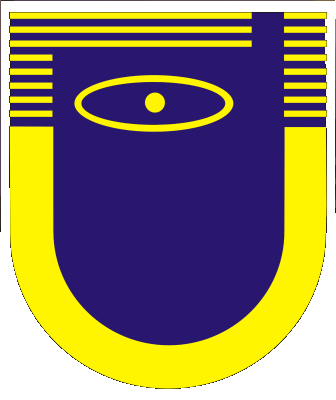Main Page
Subscription information

Current Issue
Tables of Contents
Author Index
Search

Authors
Referees

## A class of magnetic fileds which keep the self-similarity for the problems of free magnetohydrodynamic thermal convection

M. Ya. Antimirov - I. M. Volodko - O. V. Zhirnov - A. Yu. Podvysotskii

Riga Technical University, Riga LV-1000, Latvia

Abstract
In this paper the exact asymptotic solutions for the self-similar problems of the free thermal convection near a semi-infinite vertica l plate and above a wall plume in the transverse magnetic fields for Prandtl number Pr << 1 and Lykoudis number Ly -> ininity are obtained. The matched asymptotic expansions method was used. Moreover, the exact numerical solutions of these problems also were obtained for Pr = 0.01, 0.0625, 0.1, 1.0 and for such large Ly, at which the free thermal convection is fully sup-pressed (up to Ly = 20 for the vertical plate and up to Ly = 50 for the wall plume). Simultaneously the exact numerical solutions for a free thermal convection above line horizontal heat source in the similar transverse magnetic field were obtained and the region of Gray s asymptotic and approximate solutions applicability was es-timated. The new asymptotic formula was also obtained for the velocity of fluid on a vertical axis above line horizontal heat source at Pr << 1 and Ly -> infinity; this formula gives very small error for Ly >= 2 and Pr << 1. In all cases the asymptotic solutions practically coincide with the exact numerical solutions for Ly = 10 and are very close to them for Ly = 4.

Magnitnaya Gidrodinamika 34, No. 1, 3-16, 1998 [PDF, 0.71 Mb] (in Russian)
Magnetohydrodynamics 34, No. 1, 1-13, 1998 [PDF, 0.61 Mb]

Copyright: Institute of Physics, University of Latvia
Electronic edition ISSN 1574-0579
Printed edition ISSN 0024-998X
DOI: http://doi.org/10.22364/mhd